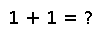# vecnorm

vecnorm(A, [p])

For any iterable container `A` (including arrays of any dimension) of numbers (or any element type for which `norm` is defined), compute the `p`-norm (defaulting to `p=2`) as if `A` were a vector of the corresponding length.

For example, if `A` is a matrix and `p=2`, then this is equivalent to the Frobenius norm.

## Examples

Checking you are not a robot: